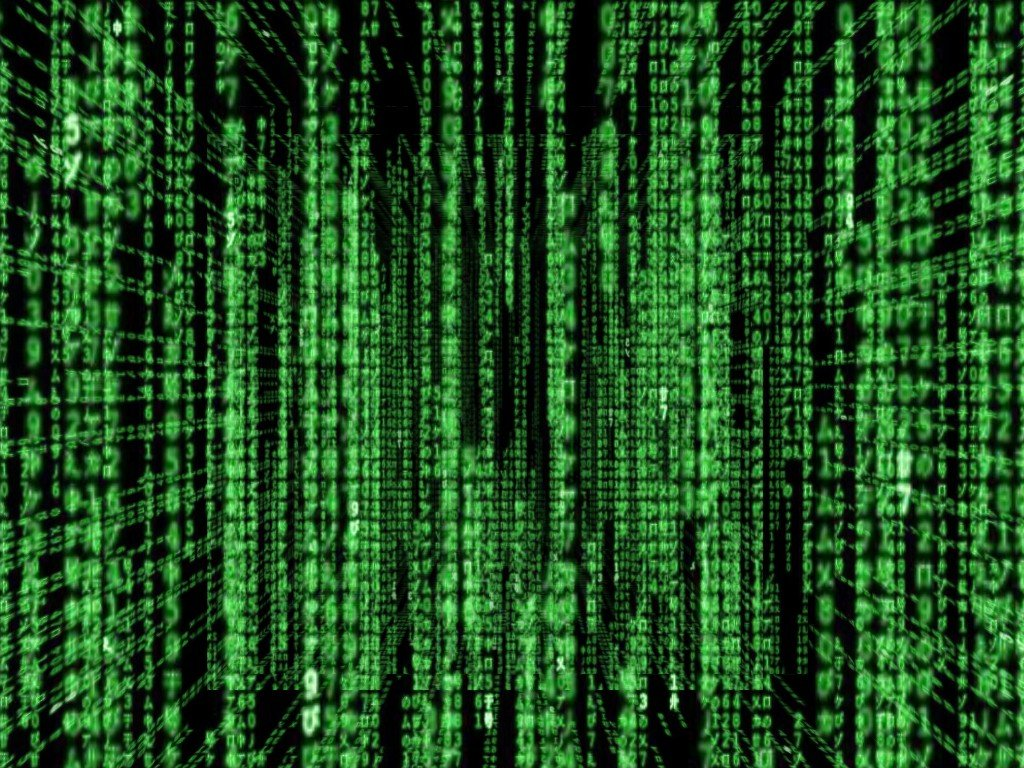# Animated backgrounds

I’d like to have random text flowing over my backgrounds, is this possible?

Yes. Several ways.

You will have to be more specific in what you are trying to achieve for us to suggest anything more though.

I’d like to have the background(The floor/skybox etc) to have matrix-like textSomething like that, except animated

Just the skybox or other textures in the scene?

In other words do you want this:Or would it just be the back wall in text?

The walls are right, but the objects wouldn’t be text

Your best bet will be to create a tiling texture with the text on it (possibly animated with a few frames) and then a custom shader to cause that text to slide.

You might want multiple textures and layer them inside the shader sliding at different speeds.

Ok, @PrivateAlpha
Lucky you,I’ve done something very similar to what you want in the past. It’s not so good but have basic idea of what you have to do.
You can test the RenderMonkey file here.

If you understand what is a “GLSL Shader”, you can make a Material with the Vertex shader shown below and add the Material to a Static Picture.[java]
varying vec2 texCoord;

void main(void)
{
gl_Position = vec4( gl_Vertex.xy, 0.0, 1.0 );
gl_Position = sign( gl_Position );

// Texture coordinate for screen aligned (in correct range):
texCoord = (vec2( gl_Position.x, - gl_Position.y ) + vec2( 1.0 ) ) / vec2( 2.0 );

}
[/java]
[java]
uniform sampler2D Font;
uniform float Time;
uniform vec2 mouse;
uniform vec2 resolution;
varying vec2 texCoord;
uniform float speed;
uniform float zoomSpeed;
uniform int layers;
uniform float layerDis;

vec4 fieldCol(vec3 pos){
return vec4(1.0);
}

void main(void)
{
vec2 uv;
vec2 blockuv;
vec4 compCol = vec4(0);
vec3 pos = vec3(0.5,0.51,Time);
for (int i=0;i<layers;i++){
uv = texCoord;
blockuv=texCoord;
// BLOCK

``````  blockuv.x =  floor((uv.x)* 30);
blockuv.y =  floor((uv.y)* 30);

//uv.y += 4.4 * blockuv.x;

blockuv.x = (blockuv.x  + 23 * i)/(i+1);
blockuv.y = (blockuv.y + 15 * i)/(i+1);

// SHIFT
uv.y += Time / speed * (1+i);

uv.x += Time / 40 + 0.4 *i;
uv += mouse / resolution;
//uv = uv * (0.1 + mod(Time / speed,layerDis) / zoomSpeed);
// ZOOM
/*
float zoom = 2 + abs(sin(0.4*blockx +Time / speed) * 2);
uv /= zoom;
*/
vec2 texuv = vec2(0);
vec2 unituv = vec2(1/30,1/30);
//texuv=uv/ (3*(1+i));
texuv += blockuv * unituv;
texuv += mod(uv / (1+(i * layerDis)),unituv);
vec4 textCol =  texture2D( Font,texuv) ;
// LIGHT
float layerLight = 1;
textCol *= (0.2 + 0.8 * sin(Time+ 0.2 * blockuv.x) * (i+1));
//textCol *= (0.8 + 0.2 * sin(Time+ 0.2 * blockuv.y) * (i+1));

compCol +=textCol;
``````

}
gl_FragColor = compCol;
}
[/java]
Thank you!